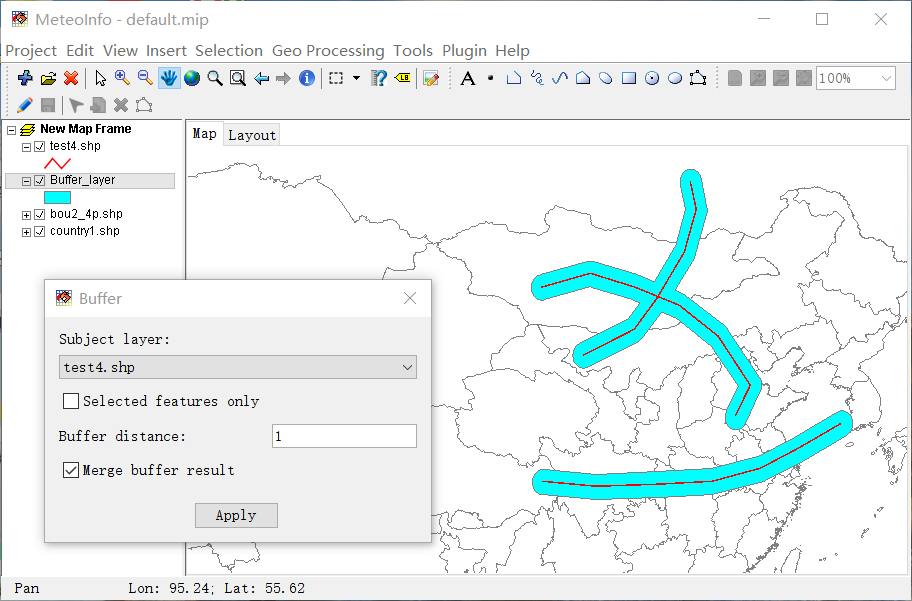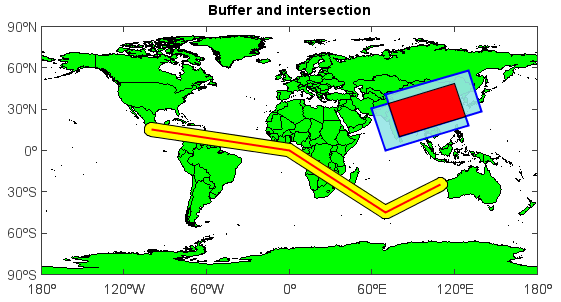# MeteoInfo 1.3.2 was released (2016-3-19)¶

MeteoInfo 1.3.2 was released. JTS Topology Suite code was included in MeteoInfo library to implement topology analysis functions.

Desktop example:MeteoInfoLab example:

import mipylib.geolib.topology as tp

axesm()
geoshow(lworld, edgecolor='k', facecolor='g')
lat = [15, 0, -45, -25]
lon = [-100, 0, 70, 110]
line1 = geoshow(lat, lon, size=2, color='r')
buf1 = tp.buffer(line1,5)
geoshow(buf1, color='y')
geoshow(lat, lon, size=2, color='r')
lat = array([30, 0, 18, 48, 30])
lon = array([60, 70, 130, 120, 60])
g1 = geoshow(lat, lon, displaytype='polygon', color=[150,230,230,230], edgecolor='b', size=2)
lat = lat + 10
lon = lon + 10
g2 = geoshow(lat, lon, displaytype='polygon', color=[150,230,230,230], edgecolor='b', size=2)
g3 = tp.intersect(g1, g2)
geoshow(g3, color='r')
#Set extent
xlim(-180, 180)
ylim(-90, 90)
xticks(arange(-180, 181, 30))
yticks(arange(-90, 91, 30))
title('Buffer and intersection')